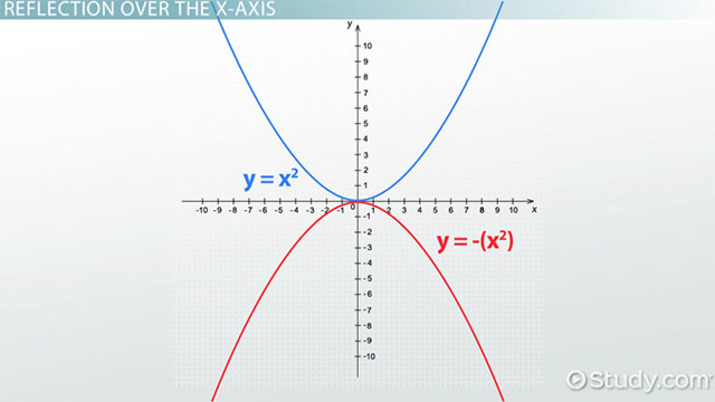# How to Reflect Quadratic Equations

Lesson Transcript
Instructor: Artem Cheprasov

Artem has a doctor of veterinary medicine degree.

In this lesson, you're going to learn about the definition of a reflection, quadratic equation, and how to visually and mathematically reflect a quartic equation over the x-axis and the y-axis.

If you ever stood in front of a mirror, or next to a calm pond, you would have seen a reflection, which in math is the flipping of a point or figure over a line of reflection (the mirror line).

Reflection can also be applied to a quadratic equation, which is simply an equation where the highest exponent is 2. The simplest of these is y = x^2, and the standard form of one is y = ax^2 + bx + c, where a, b and c are numbers and a cannot equal 0.

Let's see how we can reflect quadratic equations using graphs and some really easy math.An error occurred trying to load this video.

Try refreshing the page, or contact customer support.

Coming up next: Parabolas in Standard, Intercept, and Vertex Form

### You're on a roll. Keep up the good work!

Replay
Your next lesson will play in 10 seconds
• 0:01 Reflections &…
• 0:39 Reflection Over the X-Axis
• 1:49 Reflection Over the Y-Axis
• 3:00 Lesson Summary
Save Save

Want to watch this again later?

Timeline
Autoplay
Autoplay
Speed Speed

## Reflection Over the X-Axis

For our first example let's stick to the very simple parent graph of y = x^2.

{See video for graph}

On the screen you can see that the graph of this equation is a parabola. The reflection of such a parabola over the x-axis is simply written as y = -(x^2). In other words, the function of f(x) becomes -f(x) when reflected over the x-axis.

When reflected everything looks like an upside down version of our parent graph. Doesn't it?

{See video for graph}

In fact, remember that a positive quadratic has a smiley face, while the negative quadratic has a frown. It's really easy to recall this and important to; because, if you have an equation where the value of a is negative but you are coming up with a quadratic that is right-side up, then you know you are doing something wrong, and you need to double check where you've gone amiss.

More complex equations and graphs are really just as simple to reflect over the x-axis. Something like y = 2x^2 + 4x - 5, would become y = -(2x^2 + 4x - 5).

{See video for graph}

To unlock this lesson you must be a Study.com Member.

### Register to view this lesson

Are you a student or a teacher?